# UGC NET Psychology - Previous Years Solved Papers | Paper II November 2017

Questions
1. Match List - I with List - II and select the correct answer by choosing from the code given below :• (1) (i) (ii) (iii) (iv)
• (2) (ii) (i) (iv) (iii)
• (3) (iii) (ii) (i) (iv)
• (4) (iv) (iii) (ii) (i)

2. Which of the following is true for Tip_of_the Tongue (TOT) state ?

(a) Feeling of Knowing

(b) Feeling that memory is available

(c) Retrievable memory

(d) Maintenance recoding

Code :
• (1) (a) and (b) only
• (2) (a) and (c) only
• (3) (b) and (d) only
• (4) (c) and (d) only

3. Read each of the following two statements - Assertion (A) and Reason (R); and indicate your answer using code given below :

Assertion (A) : Conditioning done using partial reinforcement schedule decreases resistance to change.

Reason (R) : Partial reinforcement schedules influence rate of responding.

Code :
• (1) Both (A) and (R) are true, and (R) is the correct explanation of (A).
• (2) Both (A) and (R) are true, but (R) is not the correct explanation of (A).
• (3) (A) is true, but (R) is false.
• (4) (A) is false, but (R) is true.

4. Arrange the clinical scales of MMPI - II in correct sequence.
• (1) Depression → Hypochondriasis → Hysteria → Psychopathic Deviate → Masculinity-Feminity → Paranoia → Psychasthenia → Schizophrenia → Hypomania → Social Introversion
• (2) Hypochondriasis → Depression → Hysteria → Psychopathic Deviate → Masculinity-Feminity → Paranoia → Psychasthenia → Schizophrenia → Hypomania → Social Introversion
• (3) Social Introversion → Hypomania → Hypochondriasis → Depression → Hysteria → Psychopathic Deviate → Masculinity-Feminity → Paranoia → Psychasthenia → Schizophrenia
• (4) Social introversion → Hypomania → Schizophrenia → Psychasthenia → Paranoia → Masculinity-Feminity → Psychopathic deviate → Hysteria → Depression → Hypochondriasis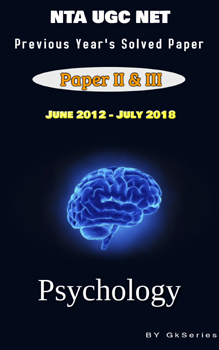#### NTA UGC NET PSYCHOLOGY E-BOOK – PREVIOUS YEAR’S SOLVED PAPERS(JUNE 2012 TO JULY 2018)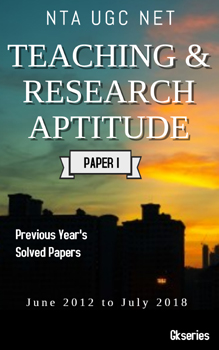#### NTA UGC NET TEACHING & RESEARCH APTITUDE E-BOOK – PREVIOUS YEAR’S SOLVED PAPERS(JUNE 2012 TO JULY 2018)

5. Allport defined personality as :
• (1) Response style
• (2) Social stimulus value
• (3) Intervening variable
• (4) Thinking style

6. Read each of the following two statements - Assertion (A) and Reason (R); and indicate your answer using code given below :

Assertion (A) : Increase in synaptic strength is due to the simultaneous stimulation of pre - synaptic and post - synaptic neurons.

Reason (R) : Long term Potentiation can be induced in the laboratory by stimulating the pre - synaptic neurons with pulses of high frequency of electricity for few seconds.

Code :
• (1) Both (A) and (R) are true, and (R) is the correct explanation of (A).
• (2) Both (A) and (R) are true, but (R) is not the correct explanation of (A).
• (3) (A) is true, but (R) is false.
• (4) (A) is false, but (R) is true.

7. Concepts allow us to identify things without _________.
• (1) Learning
• (2) Relearning
• (3) Recognition
• (4) Remembering

8. Below given are some concepts. Indicate which is the correct sequence in terms of levels of increasing abstraction ? Concepts are :

(a) Negation concept

(b) Alternative denial concept

(c) Disjunction concept

Code :
• (1) (a), (b), (c)
• (2) (a), (c), (b)
• (3) (b), (a), (c)
• (4) (c), (b), (a)

9. Match List - I with List - II and select the correct answer by choosing from the code given below :• (1) (iii) (iv) (i) (ii)
• (2) (iv) (iii) (ii) (i)
• (3) (ii) (i) (iv) (iii)
• (4) (i) (ii) (iii) (iv)

10. Binocular cues depend on :

(a) Combination of the images in the left and right retinas

(b) The way two eyes work together

(c) Image in either right or left eye

(d) Difference between the images in the two eyes

Code :
• (1) (a), (b) and (d) only
• (2) (a), (c) and (d) only
• (3) (c), (a) and (b) only
• (4) (b), (c) and (d) only

11. The correct order of the stages of Master’s and Johnson’s sexual response cycle is :
• (1) plateau, excitement, orgasm, resolution
• (2) excitement, plateau, orgasm, resolution
• (3) excitement, orgasm, plateau, resolution
• (4) excitement, plateau, orgasm, refractory

12. Where is Broca’s area located ?
• (1) Frontal Lobe
• (2) Occipital Lobe
• (3) Parietal Lobe
• (4) Temporal Lobe

13. Read each of the following two statements - Assertion (A) and Reason (R); and indicate your answer using code given below :

Assertion (A) : Generally, performance is optimal when arousal is low.

Reason (R) : On easy tasks performance becomes optimal.

Code :
• (1) Both (A) and (R) are true, (R) is the correct explanation of (A).
• (2) Both (A) and (R) are true, but (R) is not the correct explanation of (A).
• (3) (A) is true, but (R) is false.
• (4) (A) is false, but (R) is true.

14. Motivation is best understood as a state that :
• (1) Reduces a drive
• (2) Aims at satisfying a biological need
• (3) Energises an organism to act
• (4) Energises and directs behaviour

15. Match List - I with List - II and select the correct answer by choosing from the code given below :• (1) (i) (ii) (iii) (iv)
• (2) (ii) (iii) (iv) (i)
• (3) (iii) (iv) (ii) (i)
• (4) (iv) (iii) (i) (ii)

16. Match List - I with List - II and select the correct answer by choosing from the code given below :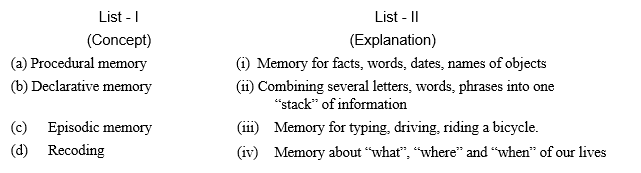• (1) (i) (ii) (iii) (iv)
• (2) (iii) (i) (iv) (ii)
• (3) (iv) (iii) (ii) (i)
• (4) (ii) (iv) (iii) (i)

17. Purposive manipulation of reinforcement contingencies, which encourage desirable behaviour, is called :
• (1) Contingency management
• (2) Contingency contracting
• (3) Contingent reinforcement
• (4) Non-contingent reinforcement

18. Which of the following depth cue is based on muscular feedback ?
• (2) Motion parallax
• (3) Accommodation

19. Nomothetic approach in personality research was mainly emphasised by :

(a) Allport

(b) Skinner

(c) Cattell

(d) Eysenk

Code :
• (1) (a) and (c) only
• (2) (a) and (b) only
• (3) (b) and (c) only
• (4) (c) and (d) only

20. Read each of the following two statements - Assertion (A) and Reason (R); and indicate your answer using code given below :

Assertion (A) : Spearman proposed the existence of specific factor of intelligence.

Reason (R) : It was observed that tetrad differences are always equal to zero.

Code :
• (1) Both (A) and (R) are true, and (R) is the correct explanation of (A).
• (2) Both (A) and (R) are true, but (R) is not the correct explanation of (A).
• (3) (A) is true, but (R) is false.
• (4) (A) is false, but (R) is true.

21. Which theory of emotion implies that every emotion is associated with a unique physiological reaction ?
• (1) James - Lange theory
• (2) Cannon - Bard theory
• (3) Two factor theory
• (4) Opponent - process theory

22. Read each of the following two statements - Assertion (A) and Reason (R); and indicate your answer using code given below :

Assertion (A) : Some people perceive wolf as dog.

Reason (R) : External features in a category of concepts are associated with each other.

Code :
• (1) Both (A) and (R) are true, and (R) is the correct explanation of (A).
• (2) Both (A) and (R) are true, but (R) is not the correct explanation of (A).
• (3) (A) is true, but (R) is false.
• (4) (A) is false, but (R) is true.

23. The frequency with which an operant response occurs before it is systematically reinforced, is called :
• (1) Rate of responding
• (2) Operant level
• (3) Orienting reflex
• (4) Autoclitic behaviour

24. An important factor which enables one to adapt to inverted vision is :
• (1) Orientation
• (2) Active movement
• (3) New learning
• (4) Frame of reference

25. ________ defined intelligence as the ability to ‘see relations and to educe correlates’.
• (1) Binet
• (2) Spearman
• (3) Terman
• (4) Boring

26. Which of the following statements are true about Eysenck Personality Questionnaire-Revised (EPQ - R) and the NEO - Personality Inventory - 3 (NEO - PI - 3) ?

(a) Both EPQ - R and NEO - PI - 3 have Extraversion and Neuroticism scales.

(b) Eysenckian Psychoticism scale correlates positively with Agreeableness, and negatively with conscientiousness scales of NEO - PI - 3.

(c) Eysenckian Psychoticism scale correlates negatively with Agreeableness and conscientiousness scales of NEO - PI - 3.

(d) Only EPQ - R has lie scale; NEO - PI - 3 does not have lie scale.

Code :
• (1) (a) and (b) only
• (2) (a) and (c) only
• (3) (a), (b) and (c) only
• (4) (a), (c) and (d) only

27. Afferent and Efferent nerves are found in which of the following ?
• (1) Sympathetic Nervous System
• (2) Para - Sympathetic Nervous System
• (3) Somatic Nervous System
• (4) Central Nervous System

28. Match List - I with List - II and select the correct answer by choosing from the code given below :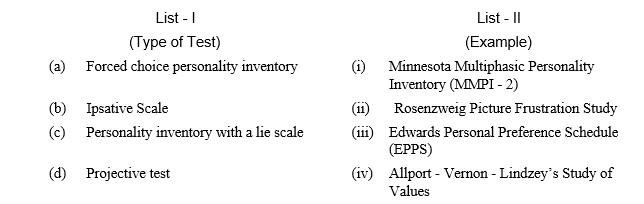• (1) (iv) (iii) (ii) (i)
• (2) (iii) (iv) (i) (ii)
• (3) (iii) (i) (iv) (ii)
• (4) (iv) (ii) (iii) (i)

29. Which is the correct sequence of parts of ear through which sound travels in the ear ?
• (1) Tymphanic membrane → Stapes → Incus → Malleus → Cochlea
• (2) Tymphanic membrane → Incus → Stapes → Malleus → Cochlea
• (3) Malleus → Incus → Stapes → Cochlea → Tymphanic membrane
• (4) Tymphanic membrane → Malleus → Incus → Stapes → Cochlea

30. The 1986 Symposium on intelligence identified the main loci of intelligence :

(a) Intelligence within the individual

(b) Intelligence within the environment

(c) Intelligence within the interaction between the individual and environment

(d) Intelligence to be measured by psychometric measures

Code :
• (1) (a) and (b) only
• (2) (c) and (d) only
• (3) (a), (b) and (c) only
• (4) (b), (c) and (d) only

31. Match List - I with List - II and select the correct answer by choosing from the code given below :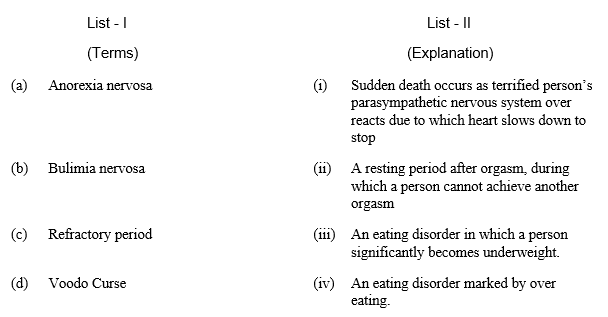• (1) (i) (ii) (iii) (iv)
• (2) (ii) (iii) (iv) (i)
• (3) (iii) (iv) (i) (ii)
• (4) (iii) (iv) (ii) (i)

32. The most commonly overlooked and obvious reason for forgetting is :
• (1) Encoding specificity
• (2) Encoding failure
• (3) Lack of sleep
• (4) Faulty eating habits

33. Which of the following sequence is correctly arranged ?
• (1) Incoming information → Rehearsal buffer → Selective attention
• (2) Rehearsal Buffer → Selective attention → Incoming information
• (3) Selective attention → Rehearsal Buffer → Incoming information
• (4) Incoming information → Selective attention → Rehearsal buffer

34. Match List - I with List - II and select the correct answer by choosing from the code given below :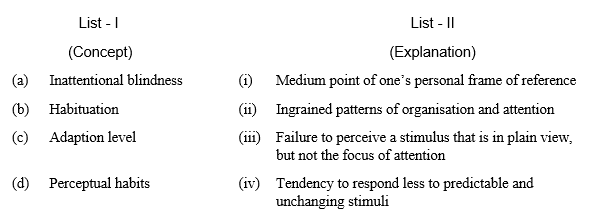• (1) (iii) (iv) (i) (ii)
• (2) (ii) (i) (iii) (iv)
• (3) (iii) (ii) (iv) (i)
• (4) (iv) (iii) (ii) (i)

35. Match List - I with List - II and select the correct answer by choosing from the code given below :• (1) (i) (ii) (iii) (iv)
• (2) (ii) (iii) (iv) (i)
• (3) (iv) (iii) (i) (ii)
• (4) (i) (iii) (iv) (ii)

36. Read each of the following two statements - Assertion (A) and Reason (R); and indicate your answer using code given below :

Assertion (A) : Some persons engage in activities such as collecting things, being good listeners, or being gullible persons.

Reason (R) : In Freudian psychoanalysis, it is assumed that fixation at oral stage results in the development of oral incorporative character.

Code :
• (1) Both (A) and (R) are true, and (R) is the correct explanation of (A).
• (2) Both (A) and (R) are true, but (R) is not the correct explanation of (A).
• (3) (A) is true, but (R) is false.
• (4) (A) is false, but (R) is true.

37. A researcher wants to scale nine attitude items by the method of paired comparison. What would be the number of pairs of the attitude items ?
• (1) 81
• (2) 72
• (3) 36
• (4) 9

38. Which of the following are regulated by Sympathetic Nervous System ?

(a) Dilation of pupils

(b) Decreased activity of Adrenal Gland

(c) Digestion is stimulated

(d) Increased Heart Rate

(e) Dryness in mouth

Code :
• (1) (a), (b) and (c) only
• (2) (b) and (c) only
• (3) (a), (d) and (e) only
• (4) (a), (c), (d) and (e) only

39. Which of the following can cause errors in reasoning ?

(a) Premise phrasing manner

(b) Foreclosure effects

(c) Confirmation bias

Code :
• (1) (a) and (b)
• (2) (a) and (c)
• (3) (b) and (c)
• (4) (a), (b) and (c)

40. Read each of the following two statements - Assertion (A) and Reason (R); and indicate your answer using code given below :

Assertion (A) : The names of objects, days of the week, the seasons and other general facts are all quite lasting and we do not forget them.

Reason (R) : Most of our basic factual knowledge about the world is almost totally immune to forgetting.

Code :
• (1) Both (A) and (R) are true and (R) is the correct explanation of (A).
• (2) Both (A) and (R) are true, but (R) is not the correct explanation of (A).
• (3) (A) is true, but (R) is false.
• (4) (A) is false, but (R) is true.

41. According to Skinner, which of the following are the characteristics of ‘Respondent Behaviour’ ?

(a) It is elicited.

(b) It is emitted.

(c) It is conditioned.

(d) It is unconditioned.

Code :
• (1) (a) and (c) only
• (2) (b) and (c) only
• (3) (a), (c) and (d) only
• (4) (b), (c) and (d) only

42. Read each of the following two statements - Assertion (A) and Reason (R); and indicate your answer using code given below :

Assertion (A) : To use a computer, we must learn to pay attention to specific stimuli, such as icons, commands and signals.

Reason (R) : Learning creates perceptual habits that affect our daily experience.

Code :
• (1) Both (A) and (R) are true and (R) is the correct explanation of (A).
• (2) Both (A) and (R) are true, but (R) is not the correct explanation of (A).
• (3) (A) is true, but (R) is false.
• (4) (A) is false, but (R) is true.

43. Arrange Thurston’s primary abilities in correct sequence :
• (1) Verbal Comprehension; Verbal Fluency; Numerical Ability; Memory; Perceptual Speed; Inductive Reasoning, Spatial Visualization
• (2) Verbal Fluency; Verbal Comprehension; Numerical Ability; Memory; Perceptual Speed; Spatial Visualization; Inductive Reasoning
• (3) Numerical Ability; Verbal Comprehension; Verbal Fluency; Memory; Inductive Reasoning; Spatial Visualization; Perceptual Speed
• (4) Spatial Visualization; Inductive Reasoning; Memory; Perceptual Speed; Numerical Ability; Verbal Fluency; Verbal Comprehension

44. Read each of the following two statements - Assertion (A) and Reason (R); and indicate your answer using code given below :

Assertion (A) : According to the Classical Test Theory (CTT), the true score component and the error component of the observed score are assumed to be uncorrelated.

Reason (R) : According to the CTT, the error component is assumed to be distributed with a mean of zero.

Code :
• (1) Both (A) and (R) are true and (R) is the correct explanation of (A).
• (2) Both (A) and (R) are true, but (R) is not the correct explanation of (A).
• (3) (A) is true, but (R) is false.
• (4) (A) is false, but (R) is true.

45. Arish, on a Wechsler type of scale, has a DIQ(deviation IQ) of 85. His percentile rank would be :46. Which one of the following conclusions can be drawn on the basis of the above study ?
• (1) The researcher’s hypothesis has been verified.
• (2) The researcher’s hypothesis has been rejected.
• (3) The researcher’s hypothesis has been partially accepted.
• (4) Inadequate data to evaluate the hypothesis.

47. In the above study, ‘association value’ can be labelled as :

(a) Continuous variable

(b) Controlled variable

(c) Organismic variable

(d) Stimulus variable

Code :
• (1) (a) and (b) only
• (2) (a) and (c) only
• (3) (a), (b) and (c) only
• (4) (a), (b) and (d) only

48. Read each of the following two statements - Assertion (A) and Reason (R); and indicate your answer using code given below :

Assertion (A) : In the above study, the subjects have been randomly assigned to the three groups.

Reason (R) : The independent variable is manipulated more effectively with random assignment of subjects.

Code :
• (1) Both (A) and (R) are true and (R) is the correct explanation of (A).
• (2) Both (A) and (R) are true, but (R) is not the correct explanation of (A).
• (3) (A) is true, but (R) is false.
• (4) (A) is false, but (R) is true.

49. Match List - I with List - II in the context of above study and select the correct answer by choosing from the code given below :• (1) (iv) (ii) (iii) (i)
• (2) (ii) (i) (iv) (iii)
• (3) (ii) (iv) (iii) (i)
• (4) (ii) (iv) (i) (iii)